# Python Learning Week 10 (Solution)

## Naïve Bayes Classification Model

Develop a python program to implement Bayesian classification model for the following dataset and classify the given test vector:

 Age Loan Class(Defaulter) 25 40000 0 35 60000 0 45 80000 0 20 20000 0 35 120000 0 52 18000 0 23 95000 1 40 62000 1 60 100000 1 48 220000 1 33 150000 1 48 142000 ?

CODE: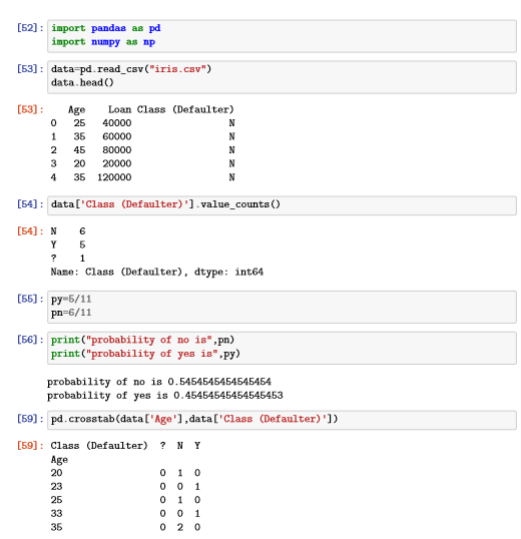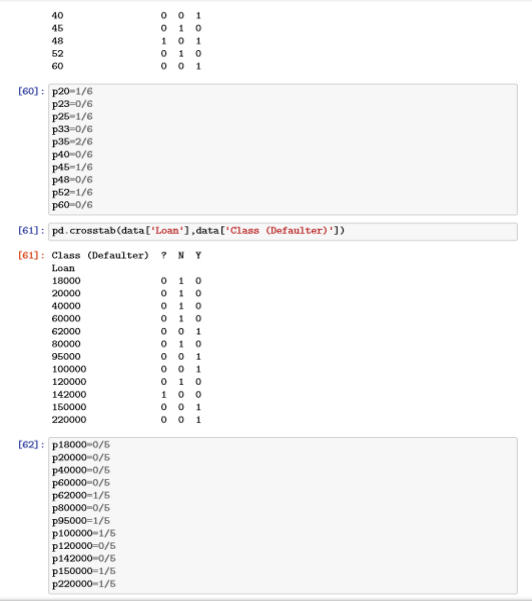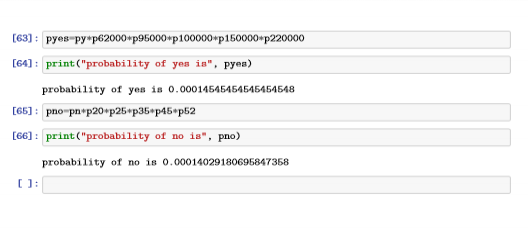Use the given cancer dataset and classify it using Bayesian classification model:

a) First create a python script and load
‘cancer’ file.

b) Identify features and classes from the

c) Perform 2-fold cross validation on the
dataset by splitting it into testing and training parts.

d) Implement a Bayesian classifier using the
above algorithm and use training dataset to classify each of the sample within
testing dataset.

e) Compute the accuracy from the predicted
test samples.

CODE: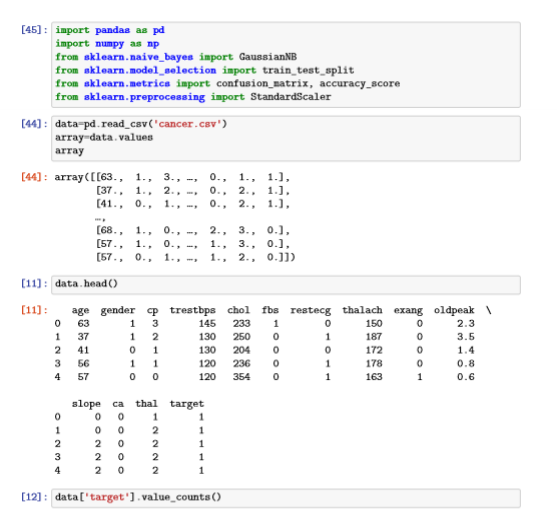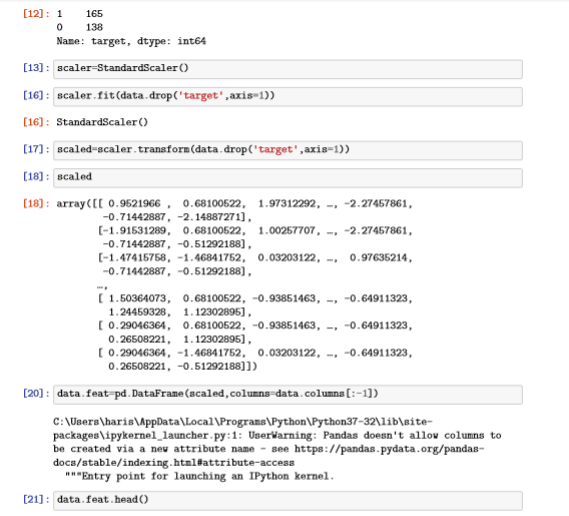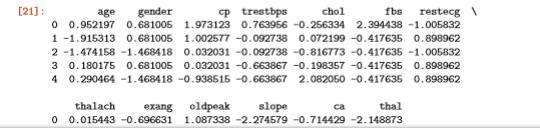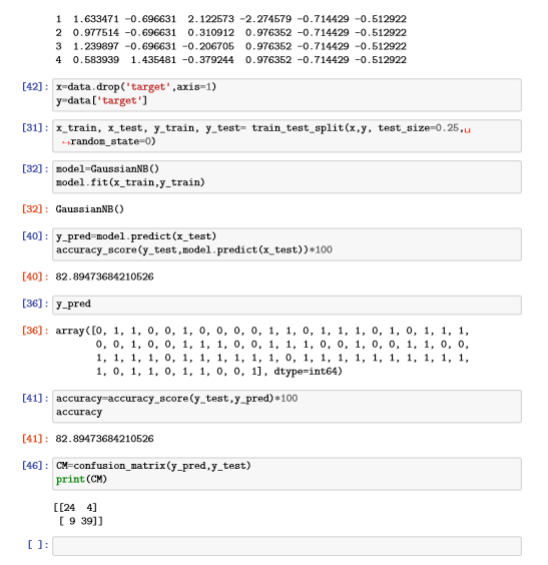What we studied in week 10:
In this week we studied Naive Bayes Classification Model. Basically Naive Bayes
classification is a probabilistic machine learning model used for classification